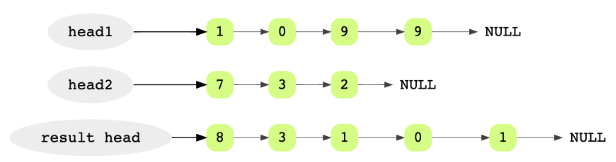## Problem Statement

Given the head pointers of two linked lists where each linked list represents an integer number (each node is a digit), add them and return the resulting linked list. Here, the first node in a list represents the least significant digit.• Handle carry

## Try it yourself

LinkedListNode* add_integers(    LinkedListNode* integer1,     LinkedListNode* integer2) {  //TODO: Write - Your - Code  return integer1;}

## Solution

// assuming both integers are stored in a linked list// e.g. 415 is stored as 5->1->4// 32 is stored as 2->3LinkedListNode* add_integers(    LinkedListNode* integer1,     LinkedListNode* integer2) {  LinkedListNode* result = nullptr;  LinkedListNode* last = nullptr;  int carry = 0;    while (      integer1 != nullptr ||      integer2 != nullptr ||      carry > 0) {    int first =         (integer1 == nullptr ? 0 : integer1->data);    int second =         (integer2 == nullptr ? 0 : integer2->data);    int sum = first + second + carry;    LinkedListNode* pNew =           new LinkedListNode(sum % 10);        carry = sum / 10;    if (result == nullptr) {      result = pNew;    } else {      last->next = pNew;    }    last = pNew;        if (integer1 != nullptr) {      integer1 = integer1->next;    }        if (integer2 != nullptr) {      integer2 = integer2->next;    }  }    return result;}int main(int argc, char* argv[]) {	vector<int> v1 = {1, 2, 3}; // 321  vector<int> v2 = {1, 2}; // 21    LinkedListNode* first = LinkedList::create_linked_list(v1);  LinkedListNode* second = LinkedList::create_linked_list(v2);  // sum should be 321 + 21 = 342 => 2->4->3  LinkedListNode* result = add_integers(first, second);  vector<int> r = {2, 4, 3}; // 342  LinkedListNode* expected = LinkedList::create_linked_list(r);  assert(LinkedList::is_equal(result, expected));  cout << endl << "First:";  LinkedList::display(first);  cout << endl << "Second:";  LinkedList::display(second);  cout << endl << "Result:";  LinkedList::display(result);  result = add_integers(first, nullptr);  assert(LinkedList::is_equal(result, first));  result = add_integers(nullptr, second);  assert(LinkedList::is_equal(result, second));}

## Solution Explanation

### Runtime Complexity

Linear, O(n)

Runtime complexity is based on the length of the linked lists.

Linear, O(n)

### Solution Breakdown

For a better understanding of the problem, let’s take a look at an example. Suppose we want to add the integers 9901 and 237. The result of this addition would be 10138.